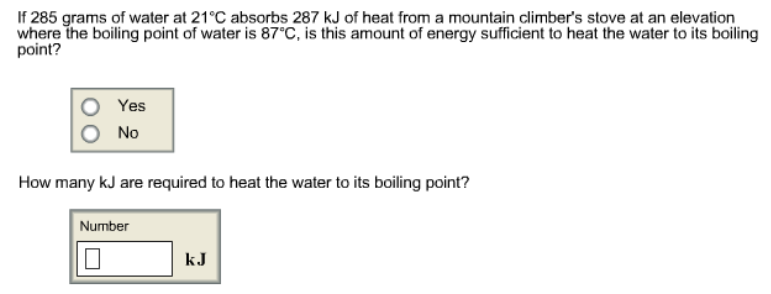Chemistry Calorimetry Solution: If 285 grams of water at 21 °C absorbs 287 kJ of h...

# Solution: If 285 grams of water at 21 °C absorbs 287 kJ of heat from a mountain climber's stove at an elevation where the boiling point of water is 87 °C, is this amount of energy sufficient to heat the water to its boiling point?           (a) Yes           (b) No How many kJ are required to heat the water to its boiling point?

Problem

If 285 grams of water at 21 °C absorbs 287 kJ of heat from a mountain climber's stove at an elevation where the boiling point of water is 87 °C, is this amount of energy sufficient to heat the water to its boiling point?

(a) Yes

(b) No

How many kJ are required to heat the water to its boiling point?View Complete Written Solution